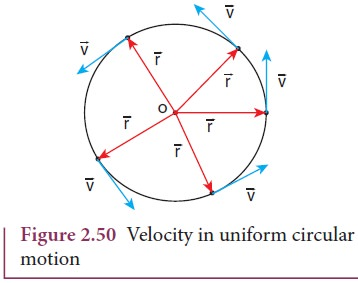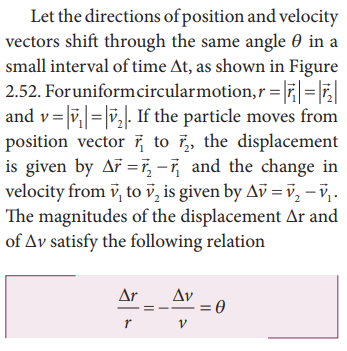Home | | Physics 11th std | Circular Motion

# Circular Motion

When a point object is moving on a circular path with a constant speed, it covers equal distances on the circumference of the circle in equal intervals of time.

Circular Motion

When a point object is moving on a circular path with a constant speed, it covers equal distances on the circumference of the circle in equal intervals of time. Then the object is said to be in uniform circular motion. This is shown in Figure 2.49.In uniform circular motion, the velocity is always changing but speed remains the same. Physically it implies that magnitude of velocity vector remains constant and only the direction changes continuously.

If the velocity changes in both speed and direction during the circular motion, we get non uniform circular motion .

## Centripetal acceleration

As seen already, in uniform circular motion the velocity vector turns continuously without changing its magnitude (speed), as shown in Figure 2.50.Note that the length of the velocity vector (blue) is not changed during the motion, implying that the speed remains constant. Even though the velocity is tangential at every point in the circle, the acceleration is acting towards the center of the circle. This is called centripetal acceleration. It always points towards the center of the circle. This is shown in the Figure 2.51.The centripetal acceleration is derived from a simple geometrical relationship between position and velocity vectors (Figure 2.48 or Figure 2.52).Here the negative sign implies that ∆v points radially inward, towards the center of the circle.For uniform circular motion v = ωr, where ω is the angular velocity of the particle about the center. Then the centripetal acceleration can be written as## Non uniform circular motion

If the speed of the object in circular motion is not constant, then we have non-uniform circular motion. For example, when the bob attached to a string moves in vertical circle, the speed of the bob is not the same at all time. Whenever the speed is not same in circular motion, the particle will have both centripetal and tangential acceleration as shown in the Figure 2.53.The resultant acceleration is obtained by vector sum of centripetal and tangential acceleration.This resultant acceleration makes an angle θ with the radius vector as shown in Figure 2.53.## Kinematic Equations of circular motion

If an object is in circular motion with constant angular acceleration α, we can derive kinematic equations for this motion, analogous to those for linear motion.

Let us consider a particle executing circular motion with initial angular velocity ω0 . After a time interval t it attains a final angular velocity ω. During this time, it covers an angular displacement θ . Because of the change in angular velocity there is an angular acceleration α.

The kinematic equations for circular motion are easily written by following the kinematic equations for linear motion in section 2.4.3

The linear displacement (s) is replaced by the angular displacement (θ ).

The velocity (v) is replaced by angular velocity (ω).

The acceleration (a) is replaced by angular acceleration (α).

The initial velocity (u) is replaced by the initial angular velocity (ω0 ).

By following this convention, kinematic equations for circular motion are as in the table given below .## Solved Example Problems for Circular Motion

### Example 2.40

A particle moves in a circle of radius 10 m. Its linear speed is given by v = 3t where t is in second and v is in m s-1.

a) Find the centripetal and tangential acceleration at t = 2 s.

b) Calculate the angle between the resultant acceleration and the radius vector.

### Solution

The linear speed at t = 2 sThe centripetal acceleration at t = 2 s isThe angle between the radius vector with resultant acceleration is given by### Example 2.41

A particle is in circular motion with an acceleration α = 0.2 rad s2.

a) What is the angular displacement made by the particle after 5 s?

b) What is the angular velocity at t = 5 s?. Assume the initial angular velocity is zero.

### Solution

Since the initial angular velocity is zero (ω0 = 0).

The angular displacement made by the particle is given byStudy Material, Lecturing Notes, Assignment, Reference, Wiki description explanation, brief detail
11th Physics : UNIT 2 : Kinematics : Circular Motion |